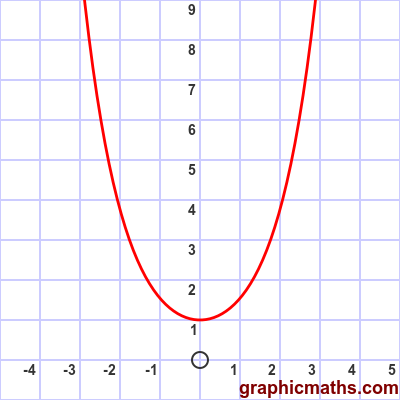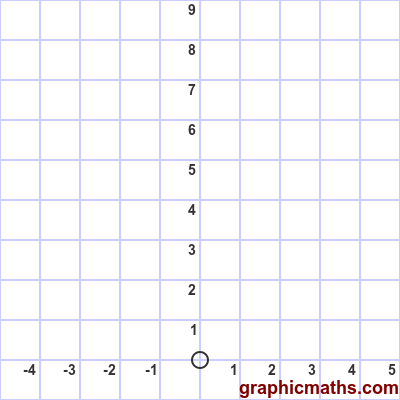# cosh function

Martin McBride, 2020-09-10
Tags cosh
Categories hyperbolic functions pure mathematics

The cosh function is a hyperbolic function. It is also known as the hyperbolic cosine function.

## Equation and graph

The cosh function is defined as:

$$\cosh{x} = \frac{e^{x}+e^{-x}}{2}$$

Here is a graph of the function:## cosh as average of two exponentials

The cosh function can be interpreted as the average of two functions, $e^{x}$ and $e^{-x}$. This animation illustrates this:## Other forms of the equation

If we multiply the top and bottom of the original equation for the cosh function by $e^{x}$ we get:

$$\sinh{x} = \frac{e^{2x}+1}{2e^{x}}$$

Alternatively, if we multiply the top and bottom of the original equation for the cosh function by $e^{-x}$ we get:

$$\sinh{x} = \frac{1+e^{-2x}}{2e^{-x}}$$

These alternative forms are sometimes useful.